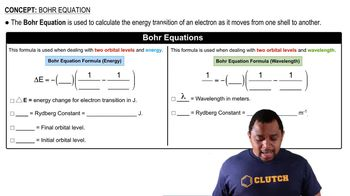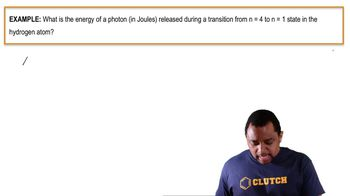Start typing, then use the up and down arrows to select an option from the list.
3:24 minutes
Problem 64
Textbook Question

# Use the Balmer equation to calculate the wavelength in nano-meters of the spectral line for hydrogen when n = 6 and m = 2. What is the energy in kilojoules per mole of the radiation corresponding to this line?Verified Solution
This video solution was recommended by our tutors as helpful for the problem above.
166views